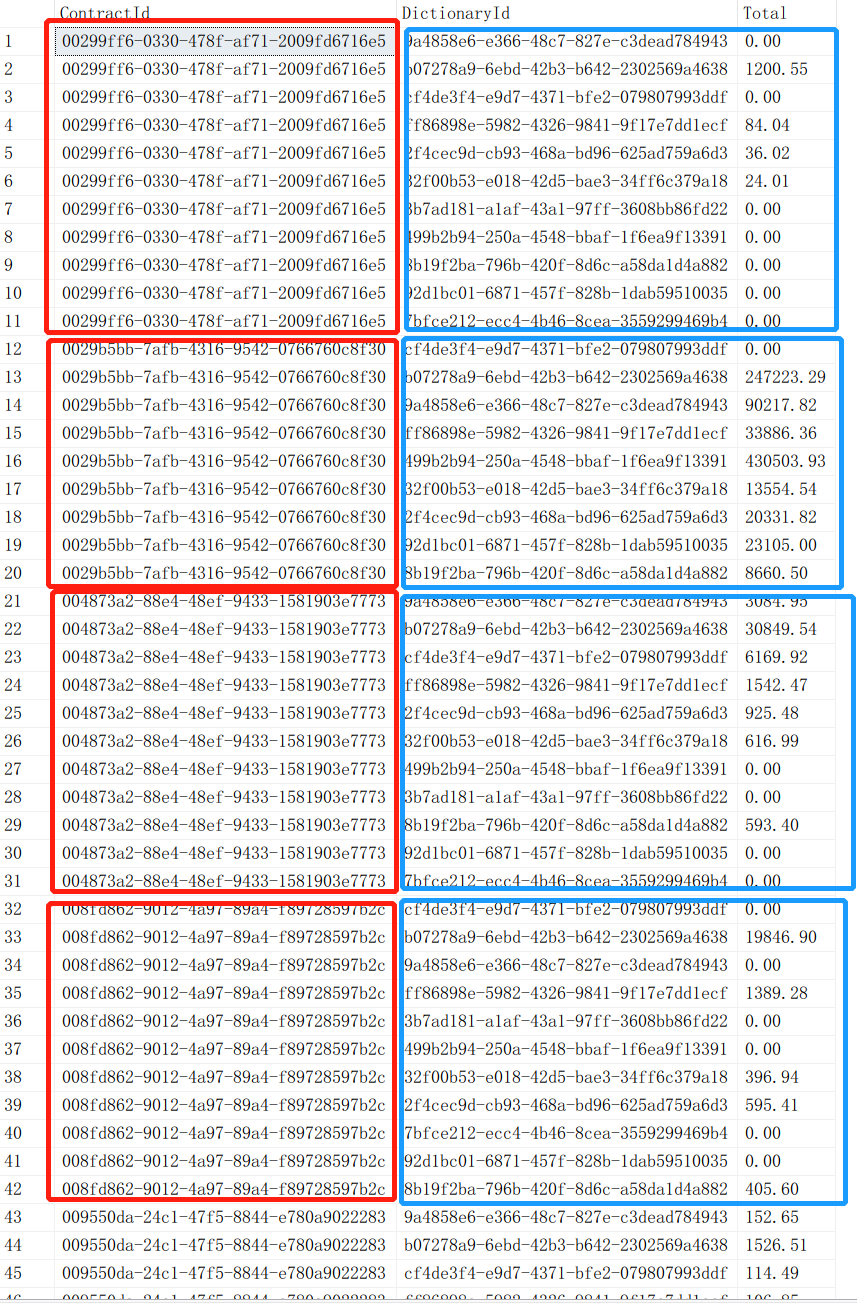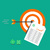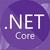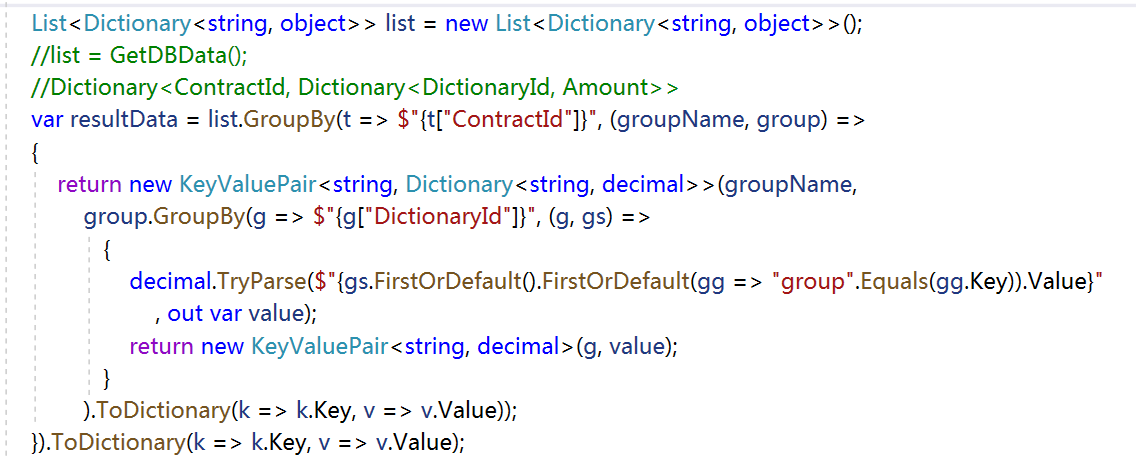# C#如何处理分组数据

Jundee 发布于 05/17 11:00Dictionary<ContractId, Dictionary<DictionaryId, Amount>>

1Dictionary> dictionary=contractBillItemVOs.GroupBy(e => e.ContractId).ToDictionary(g => g.Key, g => g.ToDictionary(b => b.DictionaryId, b => b.Amount));

0(groupName, group) =>
{
return new KeyValuePair<string, Dictionary<string, decimal>>(groupName,
group.GroupBy(g => $"{g["DictionaryId"]}", (g, gs) => { decimal.TryParse($"{gs.FirstOrDefault().FirstOrDefault(gg => "group".Equals(gg.Key)).Value}"
, out var value);
return new KeyValuePair<string, decimal>(g, value);
}
).ToDictionary(k => k.Key, v => v.Value));
}

 List<Dictionary<string, object>> list = new List<Dictionary<string, object>>();
//list = GetDBData();
//Dictionary<ContractId, Dictionary<DictionaryId, Amount>>
var resultData = list.GroupBy(t => $"{t["ContractId"]}", (groupName, group) => { return new KeyValuePair<string, Dictionary<string, decimal>>(groupName, group.GroupBy(g =>$"{g["DictionaryId"]}", (g, gs) =>
{
decimal.TryParse(\$"{gs.FirstOrDefault().FirstOrDefault(gg => "group".Equals(gg.Key)).Value}"
, out var value);
return new KeyValuePair<string, decimal>(g, value);
}
).ToDictionary(k => k.Key, v => v.Value));
}).ToDictionary(k => k.Key, v => v.Value);